# User Guide: 1 Grammar of Graphics

## Introduction

Package `ggspectra` extends `ggplot2` with stats, geoms, scales and annotations suitable for light spectra. It also defines `ggplot()` and `autoplot()` methods specialized for the classes defined in package `photobiology` for storing different types of spectral data. The `autoplot()` methods are described separately in vignette ‘Autoplot Methods’.

The new elements can be freely combined with methods and functions defined in packages `ggplot2`, `scales`, `ggrepel` and `cowplot`.

### What are `plot` specializations for?

The `plot` specializations produce fully annotated plots as `ggplot` objects, which can be further manipulated if so desired. They use the metadata stored in spectral objects of classes defined in package ‘photobiology’ to automatically generate suitable axis labels, scales and annotations. (Please, see vignette “Plot methods” for details.)

### What are `ggplot` specializations for?

The `ggplot` specializations set default `aes` according to the type of spectral object. They also add support for `unit.out` arguments allowing on-the-fly conversion of units of expression or spectral quantities. These are only defaults and can be overridden by explicit use of `aes()` to set the mapping of aesthetics.

### What are the different stats useful for?

The stats defined in this package help with with the generation of annotations and decorations of plots of spectral data. They are meant to be used only when the x aesthetic is mapped to a variable containing wavelength values expressed in nanometres. They are designed to work with spectral objects of the classes defined in package ‘photobiology’. Many of them also work well with any data frame as long as the x aesthetic mapping fulfils the expectations. Package ‘ggpmisc’ contains some equivalent stats which do not assume that x is mapped to wavelength, accepting `numeric` and datetime values.

stat default geom (used for) other uses
`stat_peaks` point (highlight maxima) wavelength label, spectral quantity label
`stat_valleys` point (highlight minima) wavelength label, spectral quantity label
`stat_label_peaks` text (wavelength label) spectral quantity label (support ‘ggrepel’)
`stat_label_valleys` text (wavelength label) spectral quantity label (support ‘ggrepel’)
`stat_find_wls` point (highlight wls at qty) wavelength label, spectral quantity label
`stat_find_qtys` point (highlight qty at wl) wavelength label, spectral quantity label
`stat_color` color, fill
`stat_wb_label` text (waveband name) rect (showing range of waveband and its color)
`stat_wb_total` text (y integral) label(s) with waveband integral
`stat_wb_mean` text (y mean) label(s) with waveband mean
`stat_wl_summary` text (y mean) label with wavelength range mean
`stat_wb_contribution` text (contribution) label(s) with waveband integral / whole spectrum integral
`stat_wb_relative` text (relative) label(s) with waveband integral / sum of integrals of all wavebands
`stat_wb_e_irrad` text (energy irradiance) rect (showing range of waveband and its color)
`stat_wb_q_irrad` text (photon irradiance) rect (showing range of waveband and its color)
`stat_wb_e_sirrad` text (spectral energy irradiance) rect (showing range of waveband and its color)
`stat_wb_q_sirrad` text (spectral photon irradiance) rect (showing range of waveband and its color)
`stat_wl_strip` rect (fill of wavelength or waveband) text (label with waveband name)
`stat_wb_box` rect (fill of waveband)
`stat_wb_hbar` errorbarh (color of waveband)
`stat_wb_column` rect (fill of waveband)

### What is `geom_spct` useful for?

The `geom_spct` geometry is a special case of `geom_area`, but with the minimum of the y range fixed to 0, but with stacking not enabled.

### What are the new scales for?

The new scales are convenience wrapper functions built on top of the scales exported by package ‘ggplot2’, but with default arguments that are suitable for spectral data.

Functions for automatic generation of secondary x-axes in the case when a variable containing wavelength data (nm) is mapped to the x aesthetic simplify the task of adding an axis with frequencies or wave numbers to the plot of a spectrum.

scale unit.exponent name labels breaks
scale_y_cps_continuous 0 cps_label() SI_pl_format()
scale_y_counts_continuous 3 counts_label() SI_pl_format()
scale_y_counts_tg_continuous 3 counts_label() SI_tg_format()
scale_y_A_internal_continuous 0 A_internal_label() SI_pl_format()
scale_y_A_total_continuous 0 A_total_label() SI_pl_format()
scale_y_Tfr_internal_continuous 0 Tfr_internal_label() SI_pl_format()
scale_y_Tfr_total_continuous 0 Tfr_total_label() SI_pl_format()
scale_y_Rfr_internal_continuous 0 Rfr_internal_label() SI_pl_format()
scale_y_Rfr_total_continuous 0 Rfr_total_label() SI_pl_format()
scale_y_s.e.irrad_continuous 0 s.e.irrad_label() SI_pl_format()
scale_y_s.q.irrad_continuous -6 s.q.irrad_label() SI_pl_format()
scale_y_s.e.response_continuous 0 s.e.response_label() SI_pl_format()
scale_y_s.q.response_continuous 0 s.q.response_label() SI_pl_format()
scale_x_wl_continuous -9 w_length_label() SI_pl_format() pretty_breaks(n=7)

In addition secondary axis definitions, `sec_axis_w_number()` and `sec_axis_w_frequency()`, and SI system formatters `SI_pl_format`, and `SI_tg_format` are exported, together with auxiliary functions for finding the nearest SI multiplier based on an arbitrary exponent.

### What are functions `color_chart()` and `black_or_white()` for?

Function `color_chart()` makes a color chart of rectangular tiles from a vector R color definitions. The chart returned is a `ggplot` object. Function `black_or_white()` accepts a vector of color definitions and returns a vector with colors `"white"` or `"black"` depending on the approximate luminosity of each color in the input. The main use is to automatically achieve suitable contrast between text plotted on top of a color background.

### What is function `autotitle()` for?

Function `autotitle()` adds a title, subtitle and/or a caption to a plot. The difference with `ggtitle()` from package ‘ggplot2’ is that `autotitle()` automatically retrieves metadata from an spectral object based on keys. It is used internally by all `autoplot()` methods defined in package ‘ggspectra’ and allowed syntax and key values are described in User Guide 2: Plot Methods together with plot annotations.

## Set up

``````library(ggplot2)
library(dplyr)
library(scales)
library(photobiology)
library(photobiologyWavebands)
library(ggspectra)
library(ggrepel)

good_label_repel <- packageVersion('ggrepel') != "0.8.0"``````

Create a collection of two source_spct objects.

``two_suns.mspct <- source_mspct(list(sun1 = sun.spct, sun2 = sun.spct / 2))``

We bind the two spectra in the collection into a single spectral object. This object includes an indexing factor, by default names `spct.idx`. We use this new object to later on demonstrate grouping in ggplots.

``two_suns.spct <- rbindspct(two_suns.mspct)``

We change the default theme.

``theme_set(theme_bw())``

## ggplot() methods for spectra

The only difference between these specializations and the base `ggplot()` method is that the aesthetics for \(x\) and \(y\) have suitable defaults. These are just defaults, so if needed they can still be supplied with a `mapping` argument with an user-defined `aes()`.

``ggplot(sun.spct) + geom_line()``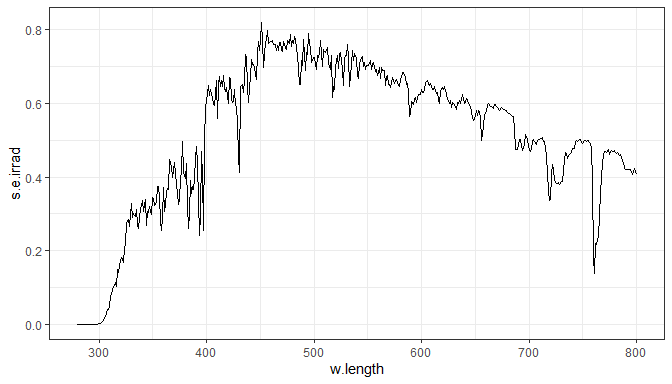It is possible to add to the defaults by means of `+` and `aes()` as shown below.

``ggplot(two_suns.spct) + aes(color = spct.idx) + geom_line()``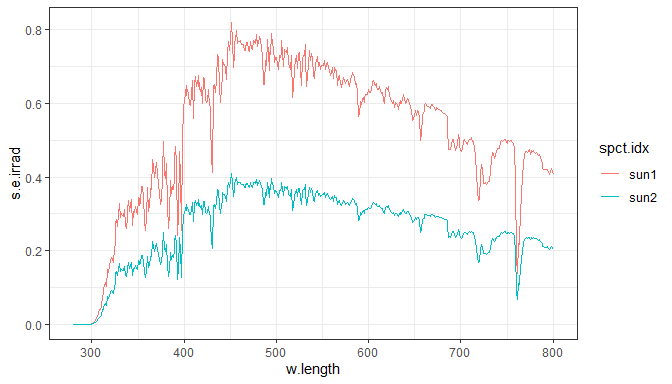If a mapping is supplied directly through `ggplot`, \(x\) and \(y\) should be included.

``ggplot(two_suns.spct, aes(w.length, s.e.irrad, color = spct.idx)) + geom_line()``In the case of `ggplot.source_spct()` an additional parameter allows setting the type of units to use in the plot. This not only sets a suitable `aes()` for \(y\) but also if needed converts the spectral data. The two possible values are `"energy"` and `"photon"` and the default, depends on option `photobiology.radiation.unit`. This parameter has a default value that can be modified through option `"photobiology.radiation.unit"`. Package ‘photobiology’ defines convenience functions for this.

``ggplot(sun.spct, unit.out = "photon") + geom_line()``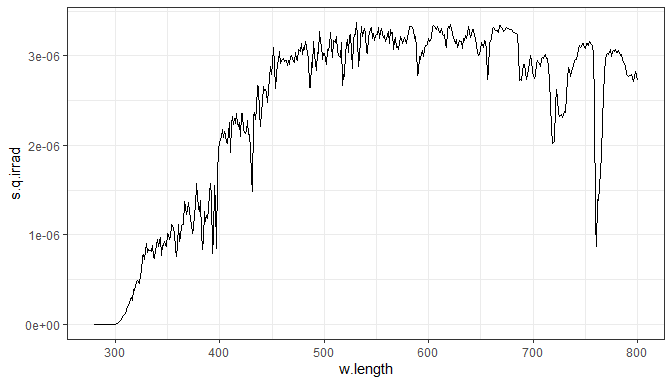After evaluation of `photon_as_default()`, a new default is in effect, but we can override it with an explicit argument if needed.

``````photon_as_default()
ggplot(sun.spct) + geom_line()
ggplot(sun.spct, unit.out = "energy") + geom_line()``````This new default will remain active for the rest of the R session, unless changed. We can easily either unset this default, or all photobiology-package related user set defaults.

``unset_user_defaults()``

The next example is for spectral properties of filters.

``ggplot(yellow_gel.spct) + geom_line()``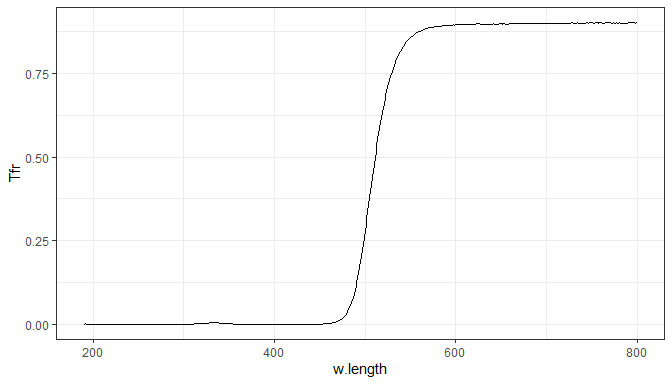In the case of `ggplot.filter_spct()` the additional parameter is called `plot.qty` and allows choosing between `"transmittance"` and `"absorbance"`.

``ggplot(yellow_gel.spct, plot.qty = "absorbance") + geom_line()``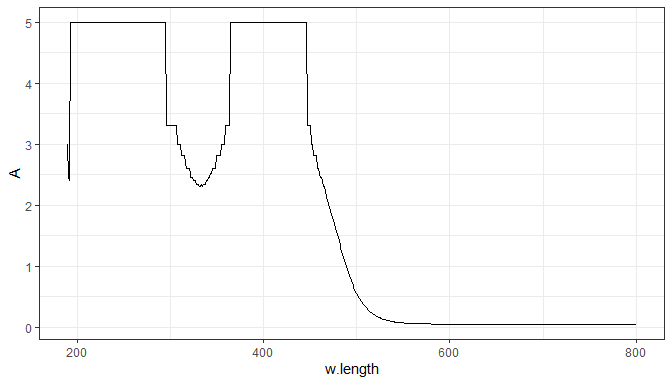In the case of `ggplot.object_spct()` three values (`"reflectance"`, `"transmittance"` and `"all"` are accepted. Passing `"all"` as argument results in the spectral data being molten into a long form, using `value` and `variable` as value and key columns, respectively. The column `variable` has three levels `Tfr`, `Rfr` and `Afr` indexing the `Tfr` and `Rfr` from the `object_spct` object plus newly calculated absorptance values.

This parameter has a default value that can be modified through option `"photobiology.filter.qty"`. Package ‘photobiology’ defines convenience functions for this.

``````Afr_as_default()
ggplot(yellow_gel.spct) + geom_line()
ggplot(polyester.spct) + geom_line()
unset_user_defaults()``````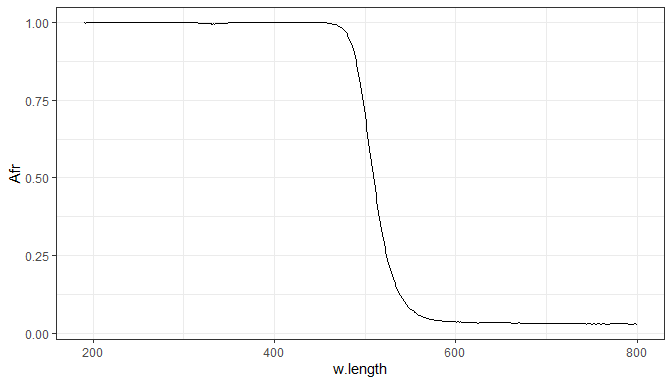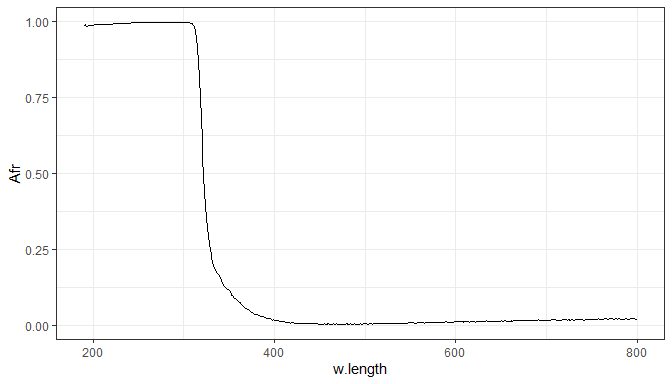The names of the additional parameters are consistent with those used in the `autoplot()` methods defined in this package.

## ggplot() methods for collections fof spectra

The `ggplot()` methods for collections of spectra work similarly to the methods for spectra when used with an spectral object containing concatenated spectra, as that shown in the previous section.

Plotting a collection of spectra using an aesthetic.

``````ggplot(two_suns.mspct) +
aes(linetype = spct.idx) +
wl_guide(ymax = -0.05) +
geom_line()``````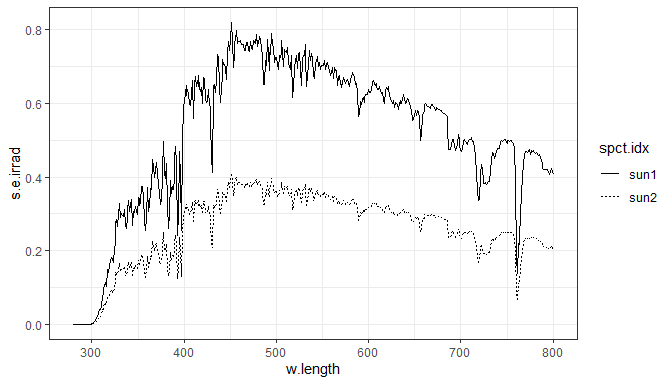Using facets.

``````ggplot(two_suns.mspct) +
wl_guide(ymax = -0.05) +
geom_spct() +
geom_line() +
facet_wrap(~spct.idx, ncol = 1L)``````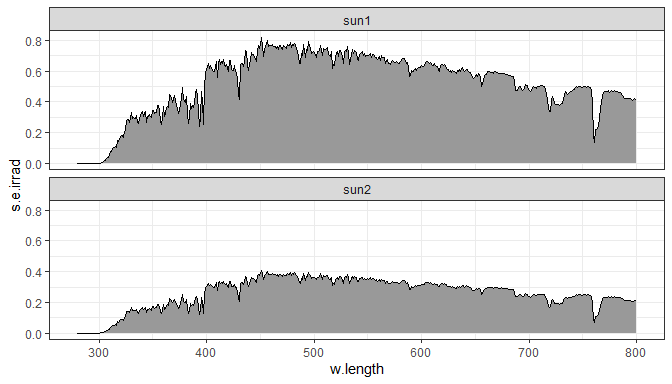``````ggplot(two_suns.mspct) +
wl_guide(ymax = -0.05) +
geom_spct() +
geom_line() +
facet_wrap(~spct.idx, ncol = 1L, scales = "free_y")``````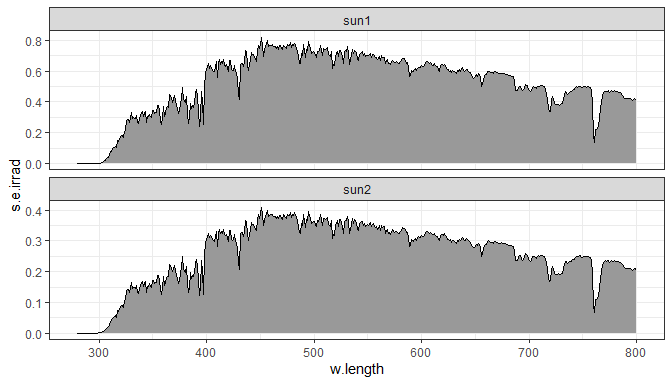## Scales

The scales provided are all wrappers of continuous scales from packages ‘ggplot2’ or ‘scales’. All pass non-specific parameters to the wrapped scales. The scales defined in ‘ggspectra’ compute suitable arguments for `name` and `labels` and pass them to the wrapped scales. The default text for the labels can be also set by the user by redefining a function, in addition than overriding the defaults in individual calls. This is a step towards multilingual support.

### Shared features

We present here, only once for all scales, examples of how to use features common to all of them. To start with we show the defaults for the scale for wavelengths and spectral energy irradiance that we will use for this examples.

``````ggplot(sun.spct) +
geom_line() +
scale_x_wl_continuous() +
scale_y_s.e.irrad_continuous()``````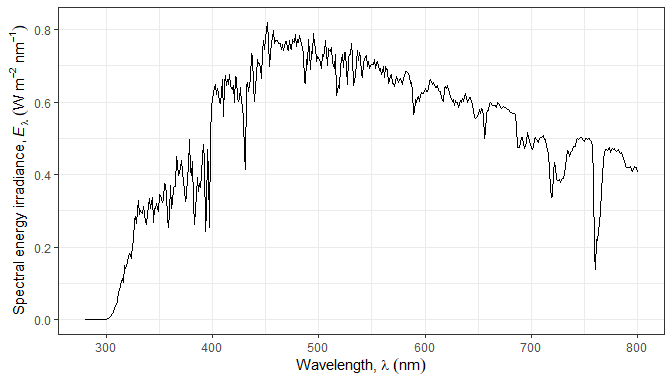Here we change the `unit.exponent` from its default value of `-9` (nanometres) to `-6` (micrometers) for wavelengts, change `0` to `-3` for irradiance. When they exist SI multipliers are used, and powers of 10 otherwise. This is not a transformation of the data, it only affects the tick labels and the axis labels in coordination. Consequently summary values will not be affected.

``````ggplot(sun.spct) +
geom_line() +
scale_x_wl_continuous(unit.exponent = -6) +
scale_y_s.e.irrad_continuous(unit.exponent = -3)``````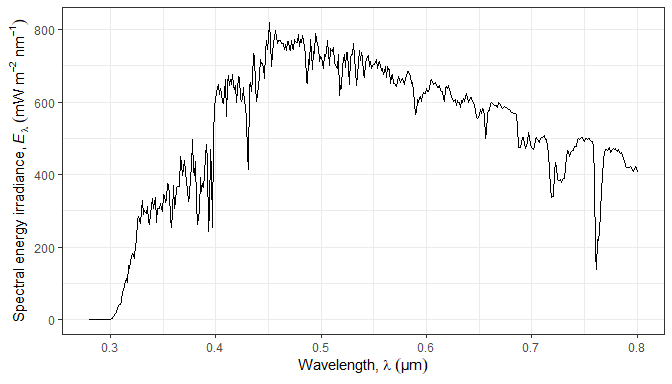``````ggplot(sun.spct) +
geom_line() +
scale_x_wl_continuous(unit.exponent = -7)  +
scale_y_s.e.irrad_continuous()``````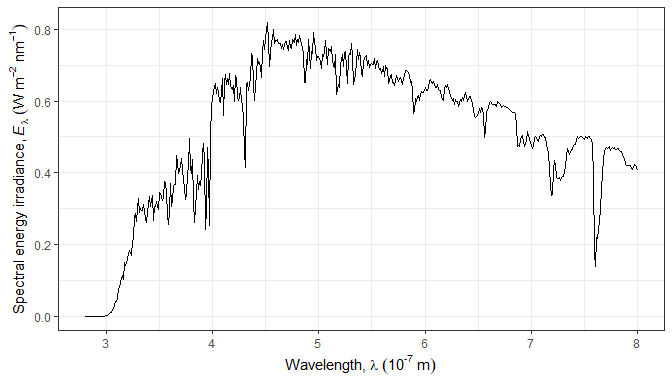We can find the largest valid SI-scaling factor that is smaller or equal to an arbitrary power of 10.

``nearest_SI_exponent(-4)``
``##  -6``

We can call this function on-the-fly.

``````ggplot(sun.spct) +
geom_line() +
scale_x_wl_continuous(unit.exponent = nearest_SI_exponent(-4))  +
scale_y_s.e.irrad_continuous()``````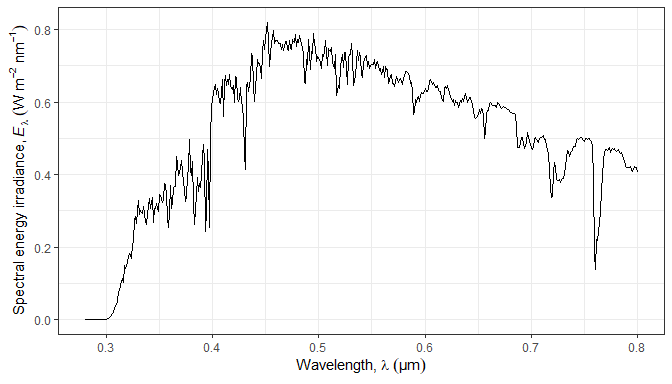In addition to formatters defined in package ‘scales’, additional formatters are defined this package, `SI_pl_format()` and `SI_tg_format()`. Most of the scales in this package use the first of them, while the second one can be passed as an argument. When it is used as shoen here, it is important to remember that as the axis label should not show scaled units, `unit.exponent` must be always passed zero as argument. I have seen this approach used in engineering related publications.

``````ggplot(sun.spct) +
geom_line() +
scale_x_wl_continuous() +
scale_y_s.e.irrad_continuous(unit.exponent = 0,
labels = SI_tg_format(exponent = -3))``````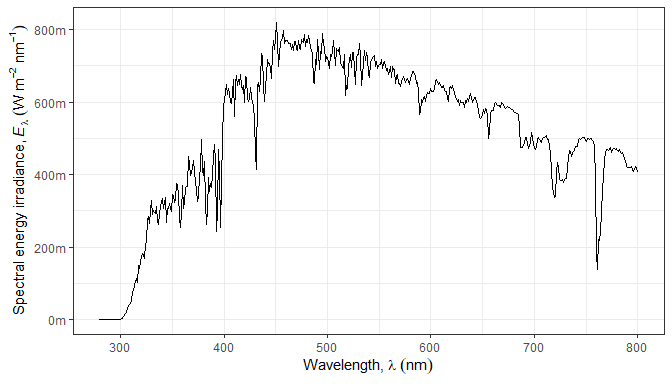Transformation objects from package ‘scales’, or user defined, can be passed as additional arguments. We replace zeros to avoid a warnings from the `log10()` call, and as the scale `limits` discards some observations, we use `na.rm = TRUE` to silence this additional warning.

``````temp.spct <- clean(sun.spct, range.s.data = c(1e-20, Inf), fill = 1e-20)
ggplot(temp.spct) +
geom_line(na.rm = TRUE) +
scale_x_wl_continuous() +
scale_y_s.e.irrad_continuous(unit.exponent = 0,
trans = "log10",
labels = trans_format("log10", math_format()),
limits = c(1e-6, NA))``````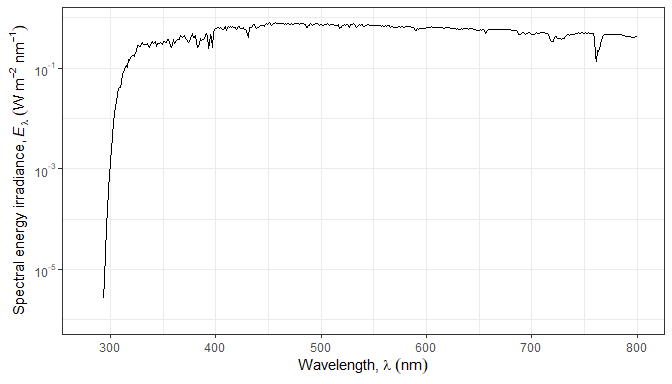The default text used can be overridden. To keep only the symbol and units pass `""` as argument (not shown).

``````ggplot(sun.spct) +
geom_line() +
scale_x_wl_continuous(label.text = "Longitud de onda,") +
scale_y_s.e.irrad_continuous(label.text = "Irradiancia,")``````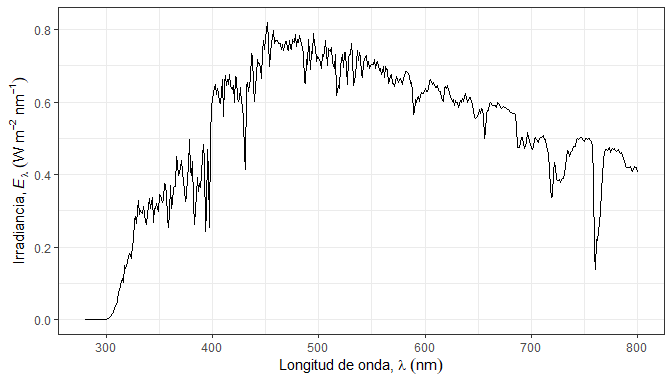If the data are normalized, we can pass the normalization wavelength as an argument.

``````norm_sun.spct <- normalize(sun.spct)
ggplot(norm_sun.spct) +
geom_line() +
scale_x_wl_continuous() +
scale_y_s.e.irrad_continuous(normalized = getNormalized(norm_sun.spct))``````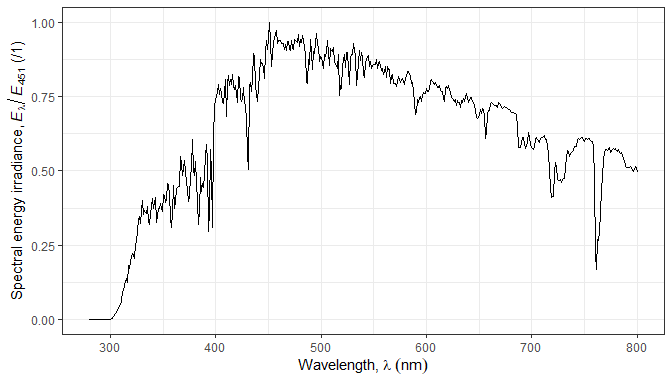If the data have been scaled, we can pass this information to the scale.

``````scaled_sun.spct <- fscale(sun.spct)
ggplot(scaled_sun.spct) +
geom_line() +
scale_x_wl_continuous() +
scale_y_s.e.irrad_continuous(scaled = is_scaled(scaled_sun.spct))``````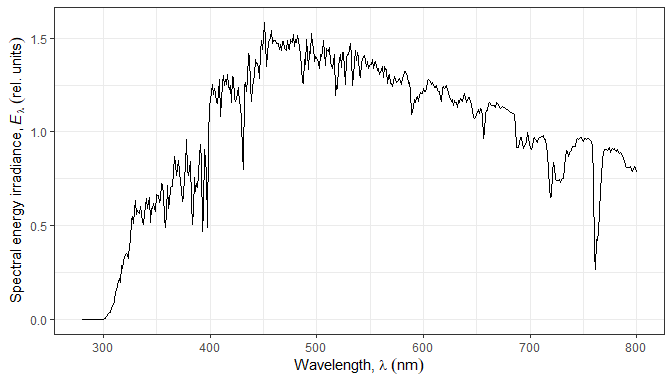Name and labels can be passed directly as arguments, but this defeats the purpose of these wrappers.

### Wavelength

Currently only one x-scale function suitable for wavelengths in nanometres is exported by package ‘ggspectra’, as well as two secondary axis definitions for wavenumber and frequency.

``````ggplot(sun.spct) +
geom_line() +
scale_x_wl_continuous()``````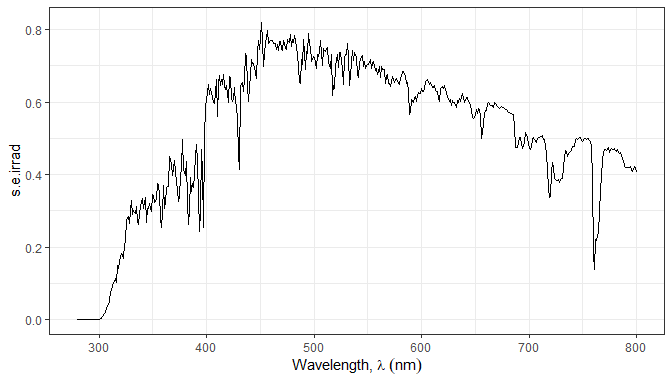Functions for automating the addition of secondary axes are available.

``````ggplot(sun.spct) +
geom_line() +
scale_x_wl_continuous(sec.axis = sec_axis_w_number())``````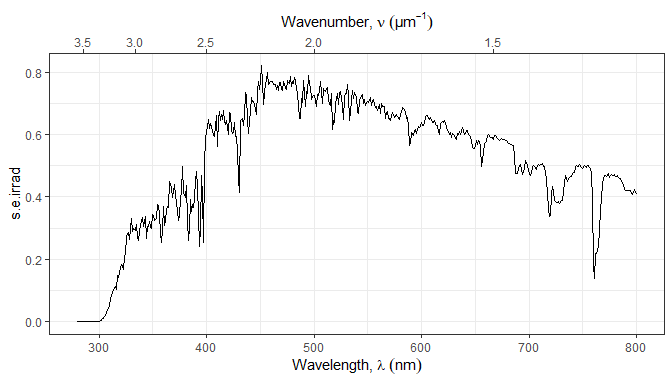``````ggplot(sun.spct) +
geom_line() +
scale_x_wl_continuous(sec.axis = sec_axis_w_frequency())``````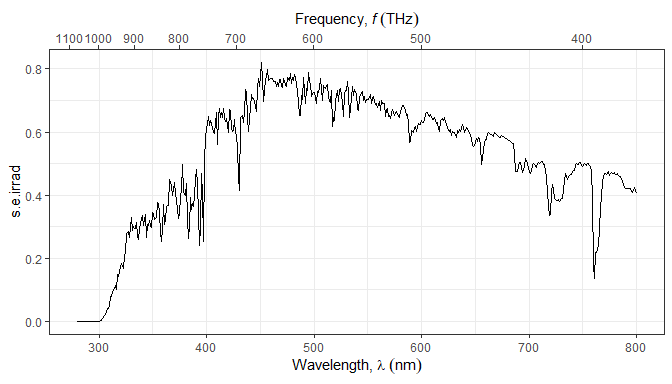As shown above for the main axis, it is possible to set a different SI scaling factor for the units in secondary scales.

``````ggplot(sun.spct) +
geom_line() +
scale_x_wl_continuous(sec.axis = sec_axis_w_frequency(15))``````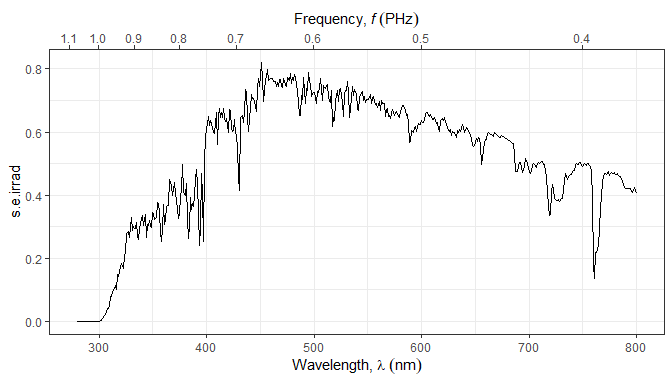### Raw counts

Raw counts from array detectors are expressed in counts, as “counts” is a whole word rather than a unit a power of ten multiplier is used.

``````ggplot(white_led.raw_spct) +
geom_line() +
scale_x_wl_continuous() +
scale_y_counts_continuous()``````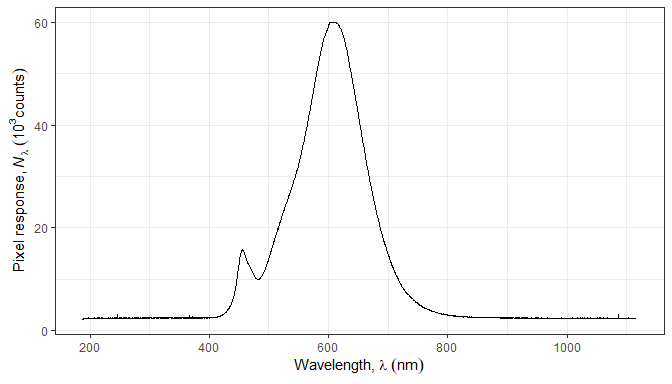The `tg` for tag version adds a suffix to the tick labels, as is common in engineering.

``````ggplot(white_led.raw_spct) +
geom_line() +
scale_x_wl_continuous() +
scale_y_counts_tg_continuous()``````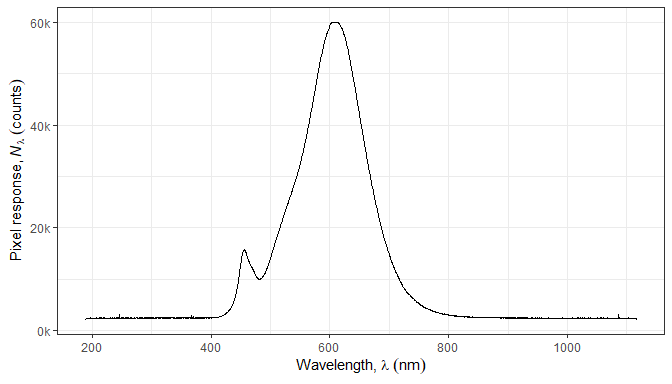### Counts per second

Raw counts from array detectors are expressed as a rate, as “counts” is a whole word rather than a unit a power of ten multiplier is used.

``````ggplot(white_led.cps_spct) +
geom_line() +
scale_x_wl_continuous() +
scale_y_cps_continuous(unit.exponent = 3)``````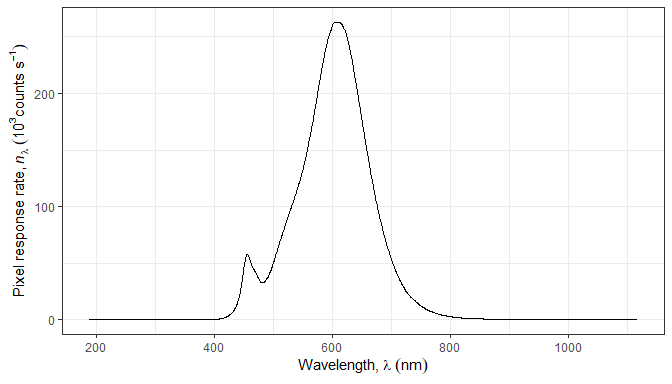### Spectral irradiance

Four scales are available, one for energy irradiance and one for photon irradiance. We show those for energy irradiance. There are equivalent scales `scale_y_s.q.irrad_continuous()` and `scale_y_s.e.irrad_log10()` for photon irradiance.

``````ggplot(sun.spct) +
geom_line() +
scale_x_wl_continuous() +
scale_y_s.e.irrad_continuous()````````````ggplot(sun.spct, unit.out = "photon") +
geom_line() +
scale_x_wl_continuous() +
scale_y_s.e.irrad_log10(unit.exponent = -6)``````
``## Warning: Transformation introduced infinite values in continuous y-axis``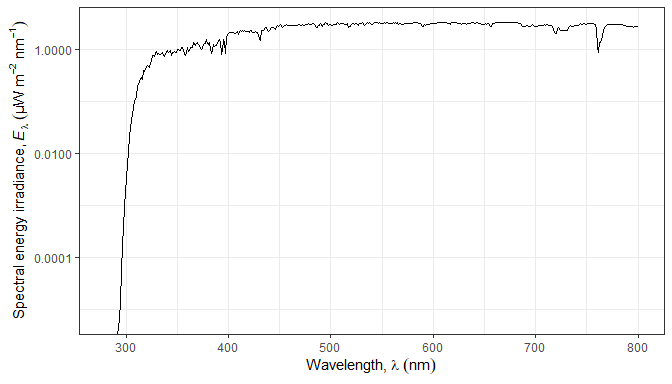### Response and action

Four scales are available, for energy response and action and for photon response and action. We show those for energy irradiance. There are equivalent scales `scale_y_q.e.resoponse_continuous()` and `scale_y_s.q.action_continuous()` for photon irradiance.

The difference between response and action spectra stems from the measurement procedure. We will wrongly use here response data for both examples.

``````ggplot(ccd.spct) +
geom_line() +
scale_x_wl_continuous() +
scale_y_s.e.response_continuous(unit.exponent = 6)``````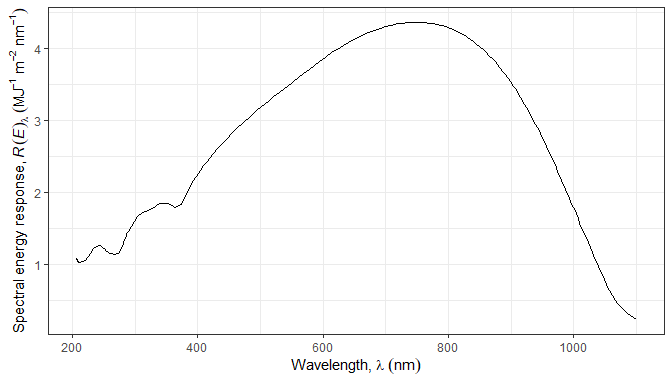The figures are identical, but the text and symbol on the y-axis label are different.

``````ggplot(ccd.spct) +
geom_line() +
scale_x_wl_continuous() +
scale_y_s.e.action_continuous(unit.exponent = 6)``````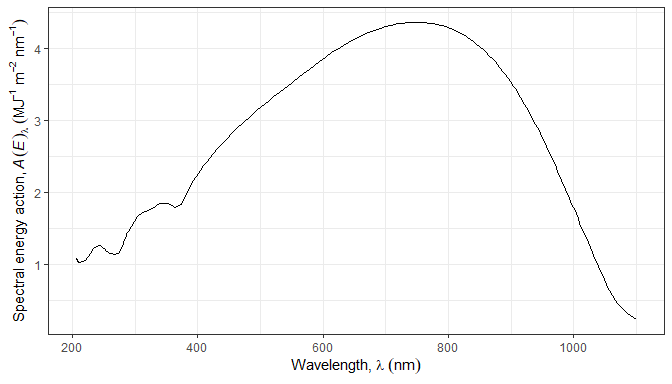### Transmittance

Two definitions of transmittance exist, total and internal. To obtain the correct labels we query the object containing the data. If we plot data from a data frame or tibble, then we can manualy pass one of `"total"` or `"internal"` as argument.

``````ggplot(yellow_gel.spct) +
geom_line() +
scale_x_wl_continuous() +
scale_y_Tfr_continuous(Tfr.type = getTfrType(yellow_gel.spct))``````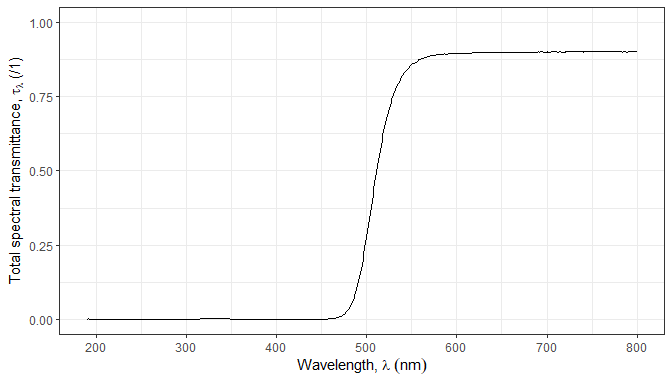``````ggplot(yellow_gel.spct) +
geom_line() +
scale_x_wl_continuous() +
scale_y_Tfr_continuous(Tfr.type = getTfrType(yellow_gel.spct),
labels = percent)``````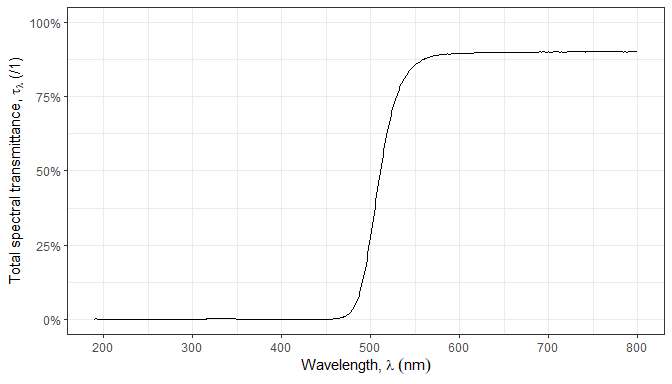``````gel_internal.spct <- convertTfrType(yellow_gel.spct, Tfr.type = "internal")
ggplot(gel_internal.spct) +
geom_line() +
scale_x_wl_continuous() +
scale_y_Tfr_continuous(Tfr.type = getTfrType(gel_internal.spct))``````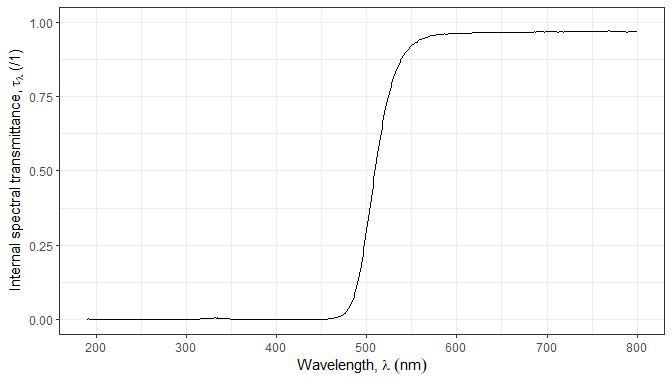### Absorbance

Two definitions of absorbance exist, total and internal. To obtain the correct labels we query the object containing the data. If we plot data from a data frame or tibble, then we can manualy pass one of `"total"` or `"internal"` as argument. This package only supports absorbance as defined using logs on base 10.

``````ggplot(gel_internal.spct, plot.qty = "absorbance") +
geom_line() +
scale_x_wl_continuous() +
scale_y_A_continuous(Tfr.type = getTfrType(gel_internal.spct))``````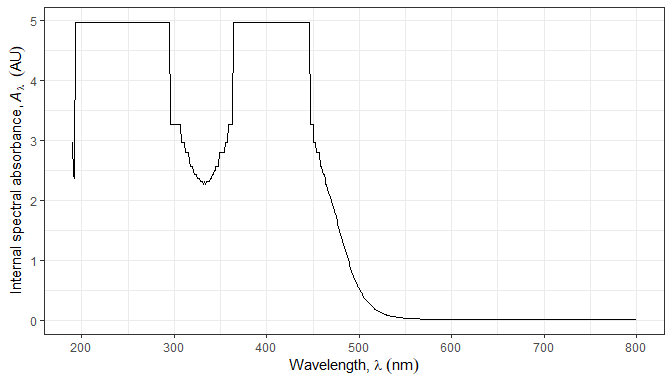### Absorptance

Absorptance has only one definition, at least within this package.

``````ggplot(yellow_gel.spct, plot.qty = "absorptance") +
geom_line() +
scale_x_wl_continuous() +
scale_y_Afr_continuous()``````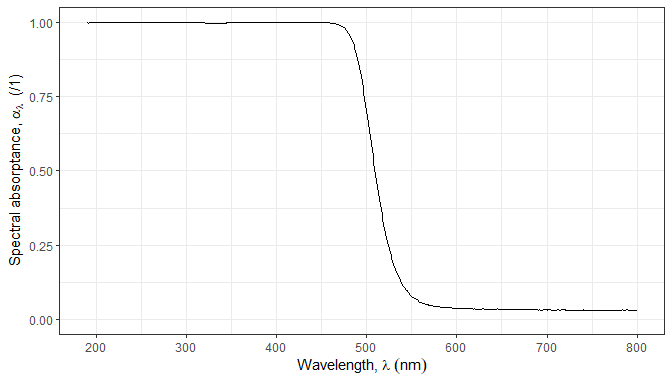### Reflectance

Two definitions of reflectance exist, total and specular. To obtain the correct labels we query the object containing the data. If we plot data from a data frame or tibble, then we can manualy pass one of `"total"` or `"specular"` as argument.

``````ggplot(green_leaf.spct) +
geom_line() +
scale_x_wl_continuous() +
scale_y_Rfr_continuous(Rfr.type = getRfrType(green_leaf.spct))``````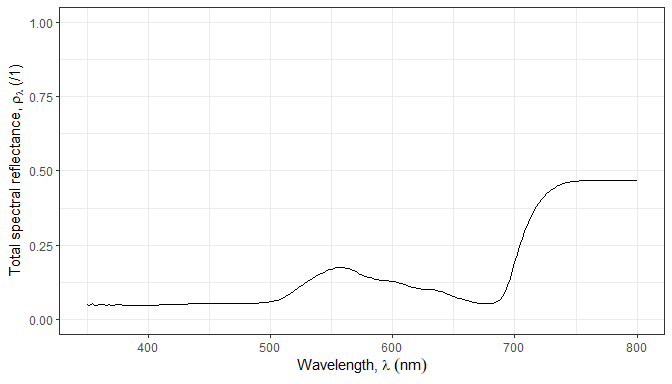## Stats

Several `ggplot` stats are defined by this package. All of them target light spectra, as they expect \(x\) to represent wavelengths expressed in nanometres. However, they should behave correctly as long as this is true, with any `ggplot` object, based on any data format acceptable to `ggplot`. The name of the original variable is irrelevant, and it is the user responsibility to supply the correct variables through `aes()`. Of course, when using the spectral classes defined in package `photobiology` the defaults easy this task.

### Peaks, valleys and target values

Four stats are available for peaks and valleys, with the same formal parameters. These stats do not fit peaks, simply search for local maxima and local minima in the data as supplied. Stats `stat_peaks()` and `stat_valleys()` subset the original data while `stat_label_peaks()` and `stat_label_valleys()` only set a boolean flag to mark the local extremes. Two stats are available for highlighting arbitrary locations in spectra, one of them, `stat_find_wls()` accepts a target for the spectral quantity and locates the corresponding wavelength values while the other, `stat_find_qtys()` accepts a target wavelength value and locates the corresponding spectral quantity value. Stats `stat_find_wls()` and `stat_find_qtys()` subset the original data or generate new data by interpolation.

All six stats set the same default aesthetics based on calculated values. Not all of these default aesthetics are used by the default geom, but they make using other geoms easier. Furthermore generated text labels are formatted with `sptintf()` and these six stats accept format definitions through parameters `label.fmt`, `x.label.fmt`, and `y.label.fmt`. Please see the documentation for a list of all the computed varaibles returned in `data`. These stats use internally functions `photobiology::find_peaks()` and `photobiology::find_wls()` and arguments are passed down to them.

The examples that follow, apply with minimal changes to `stat_peaks()`, `stat_valleys()`, `stat_find_wls()` and `stat_find_qtys()`.

``ggplot(sun.spct) + geom_line() + stat_peaks(color = "red")``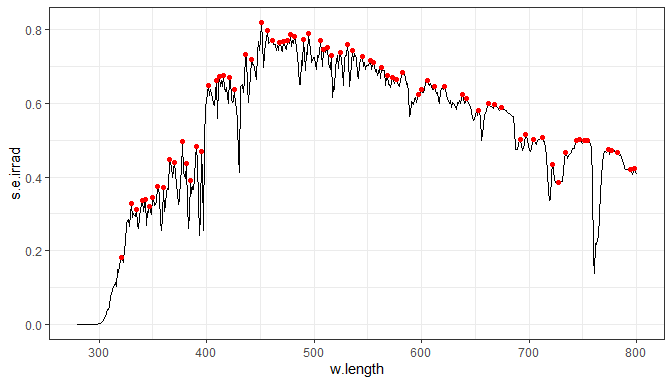Because of the conversion, the location of maxima and minima when an irradiance or response spectrum is expressed in photon- vs. energy-based units may differ. This is expected and not a bug.

``ggplot(sun.spct, unit.out = "photon") + geom_line() + stat_peaks(color = "red")``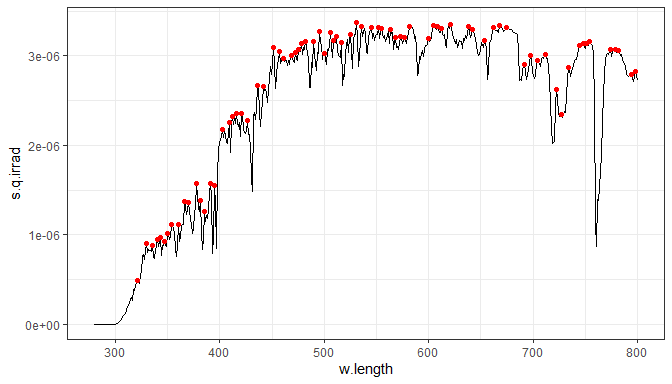The complement to `stat_peaks()` is `stat_valleys`.

``ggplot(sun.spct) + geom_line() + stat_valleys(color = "blue")``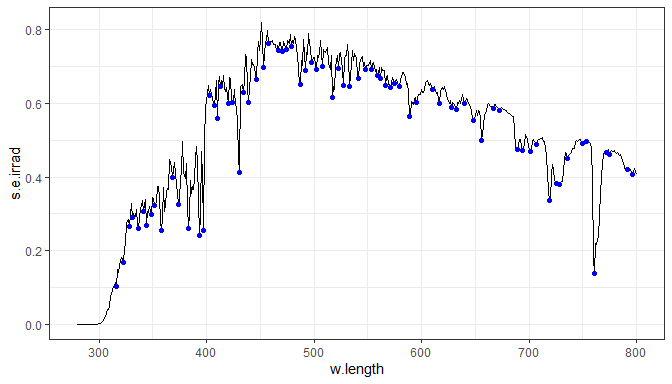Stats `stat_find_wls()` and `stat_find_qtys()` are another complementary pair. The default target, used in this example, is the half maximum.

``ggplot(yellow_gel.spct) + geom_line() + stat_find_wls(color = "darkgreen")``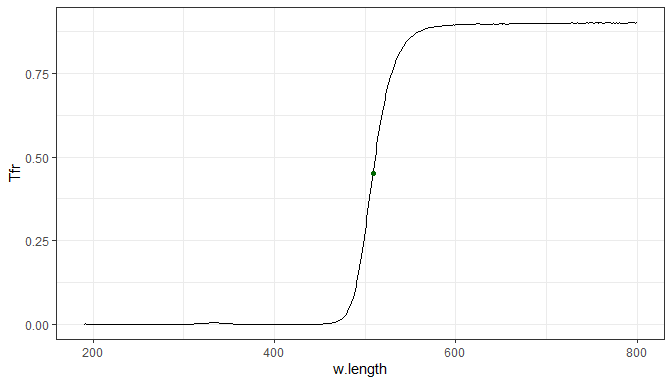One of the values calculated and mapped is colour as seen by humans corresponding to the wavelength at the location of peaks and valleys. The colour is mapped to the `fill` aesthetic, so using a ‘filled’ shape results in a colourful plot. The identity scale is needed so that the correct colours are displayed using the colour definitions in the calculated data instead of a palette.

``````ggplot(sun.spct) + geom_line() +
stat_peaks(shape = 21, color = "black") + scale_fill_identity()``````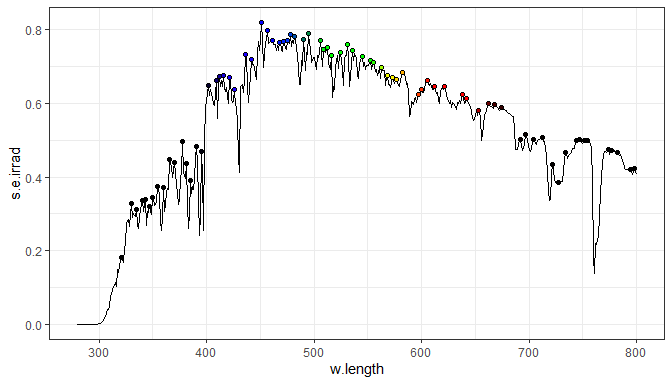We can use any of the aesthetics affecting the default geom, `"point"`, and also with other _geom_s.

``````ggplot(sun.spct) + geom_line() +
stat_peaks(span = 21, shape = 4, color = "red", size = 2) +
stat_peaks(span = 21, color = "red", geom = "rug", sides = "b")``````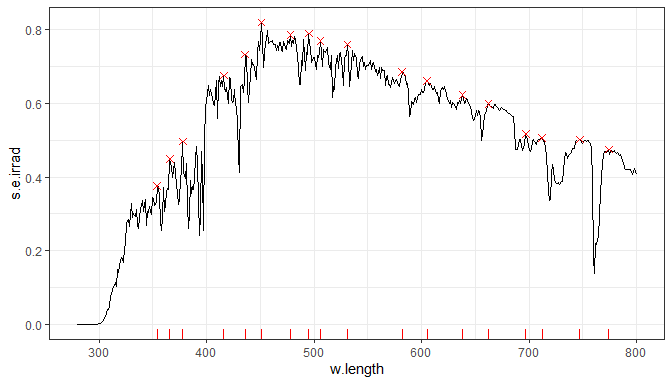We can use several other geoms as needed, demonstrated here with geom `"text"`.

``````ggplot(sun.spct) + geom_line() +
stat_peaks(span = 21, geom = "text", color = "red", vjust = "bottom") ``````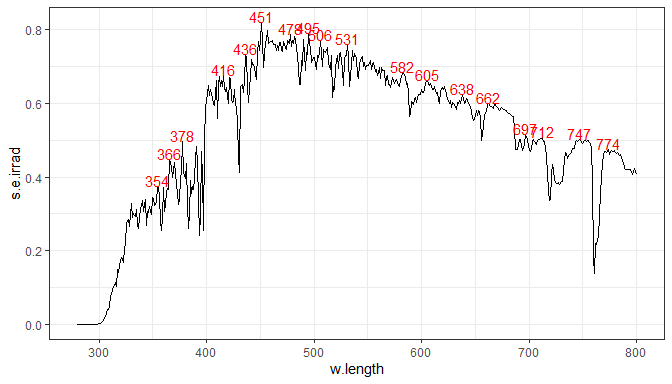The same stat can be included more than once in the same plot, using different geoms. We here in addition demonstrate the use of several different parameters. The `span` argument determines the number of consecutive observations tested when searching for a local extreme, and it should be an odd integer number. In addition we here demonstrate the use of a geom new to `ggplot2` 2.0.0 called `"label"` which again results in colourful labels by default. Here we make use of the computed variable `BW.color` which is set to `"white"` or `"white"` for maximum contrast with the computed variable `fill`.

``````ggplot(sun.spct) + geom_line() +
stat_peaks(shape = 21, span = 25, size = 2) +
scale_fill_identity() +
stat_peaks(aes(color = ..BW.color..),
geom = "label", span = 25, vjust = "bottom", size = 3) +
scale_color_identity()``````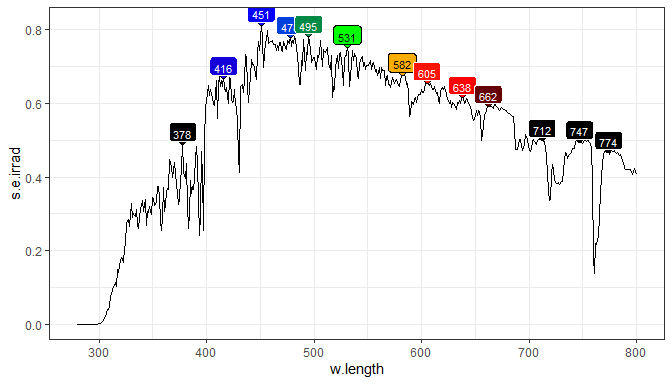To avoid overlap between labels we can use the repulsive geoms from package ‘ggrepel’. In this case we need to use `stat_label_peaks()`. Repulsion allows the use of larger labels. We also need to expand the \(y\) limits to make space for the labels. Here we also demonstrate the arguments passed to the geom for a nice display.

``````ggplot(sun.spct) + geom_line() +
stat_peaks(shape = 21, span = 25, size = 2) +
stat_label_peaks(geom = "label_repel", span = 41,
segment.color = "black",
size = 3, vjust = 1, nudge_y = 0.1,
direction = "y") +
stat_valleys(shape = 21, span = 25, size = 2) +
stat_label_valleys(geom = "label_repel", span = 51,
segment.color = "black",
size = 3, vjust = 0, nudge_y = -0.1,
direction = "y") +
scale_fill_identity() + scale_color_identity() +
expand_limits(y = c(-0.08, 0.9))``````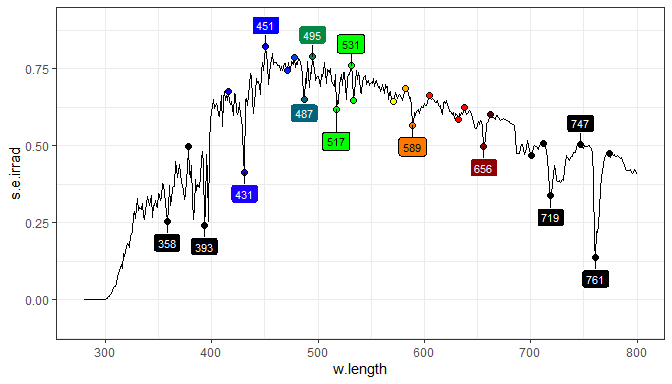If we do not use a repulsive geom that skips labels set to `""`, the plot produced by `stat_label_peaks` is of little use unless we explicitly skip the unlabelled observations as shown below.

``````ggplot(sun.spct) + geom_line() +
stat_peaks(shape = 21, span = 25, size = 2) + scale_fill_identity() +
stat_label_peaks(geom = "label", span = 25, size = 3, na.rm = TRUE) +
scale_color_identity() +
expand_limits(y = c(NA, 0.9))``````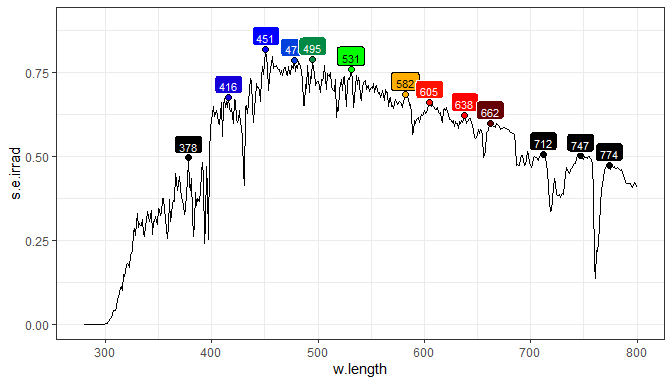Setting `span` to `NULL` results in the span set to the range of the data, and so in this case the stat returns the global extreme, instead of a local one. In this case we use geoms `"vline"` and `"hline"` taking advantage that suitable aesthetics are set by the stats.

``````ggplot(sun.spct) + geom_line() +
stat_peaks(span = NULL, geom = "vline", linetype = "dotted", color = "red") +
stat_peaks(span = NULL, geom = "hline", linetype = "dotted", color = "red")``````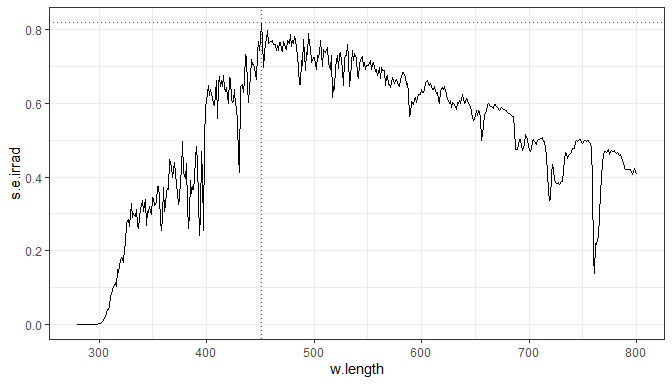By default the `label` aesthetic is mapped to a calculated label `x.label` giving the wavelength in nanometres. This mapping can be changed to give to a label giving the y-value at the peak.

``````ggplot(sun.spct) + geom_line() +
stat_label_peaks(aes(label = stat(y.label)),
span = 31, geom = "label_repel", size = 3,
label.fmt = "y = %1.2f", segment.colour = "red",
min.segment.length = unit(0.05, "lines"),
nudge_y = 0.1) +
expand_limits(y = 1) +
scale_fill_identity() + scale_color_identity()``````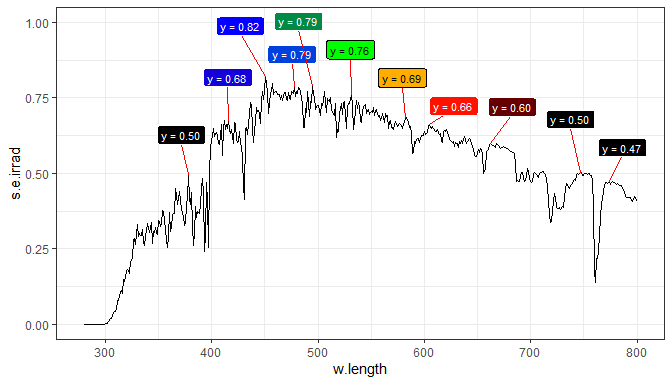As aesthetics can use values computed on-the-fly we can even use `paste()` to map a label that combines both values and adds additional text.

``````ggplot(sun.spct) + geom_line() +
stat_peaks(span = NULL, color = "red") +
stat_peaks(span = NULL, geom = "text", vjust = -0.5, color = "red",
aes(label = paste(stat(y.label), "at", stat(x.label), "nm"))) +
expand_limits(y = c(NA, 0.9))``````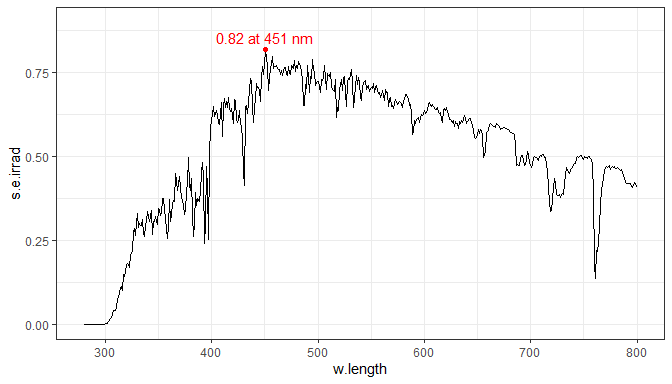Finally we demonstrate that both stats can be simultaneously used. One can also choose to use different spans as demonstrated here, resulting in more maxima being marked by points than labelled with text.

``````ggplot(sun.spct) + geom_line() +
stat_peaks(span = 21, geom = "point", colour = "red") +
stat_valleys(span = 21, geom = "point", colour = "blue") +
stat_peaks(span = 51, geom = "text", colour = "red",
vjust = -0.3, label.fmt = "%3.0f nm") +
stat_valleys(span = 51, geom = "text", colour = "blue",
vjust = 1.2, label.fmt = "%3.0f nm")``````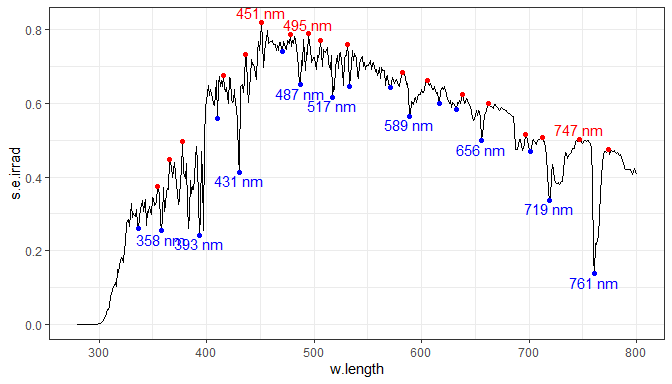This final example shows a few additional tricks used in this case to mark and label the maximum of the spectrum. This example also demonstrates why it is important that these are stats. The peaks are searched and labels generated once for each group, in this case each facet.

``````ggplot(two_suns.spct) + aes(color = spct.idx) +
geom_line() + ylim(NA, 0.9) +
stat_peaks(span = NULL, color = "black") +
stat_peaks(span = NULL, geom = "text", vjust = -0.5, size = 3,
color = "black",
aes(label = paste(stat(y.label), "at", stat(x.label), "nm"))) +
facet_grid(spct.idx~.)``````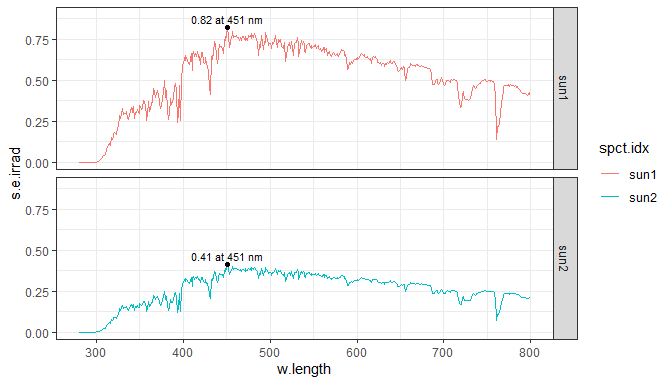An additional statistics, `stat_spikes()`, returns the same computed variables as `stat_peaks()` and `stat_valleys()` but detects only very narrow peaks and valleys, usually called spikes. They are common in Raman spectra but can also appear occasionally in any measurement with array spectrometers when integration times are long or if some pixels in an array detector are defective (e.g., hot pixels and dead pixels). Usually spikes are considered “noise” to be removed, but occasionally we may want to highlight in a plot the spikes, and do this consistently with function `despike()` from package ‘photobiology’.

``````ggplot(white_led.raw_spct, aes(w.length, counts_3)) +
geom_line() +
stat_spikes(color = "red", z.threshold = 8, max.spike.width = 7)``````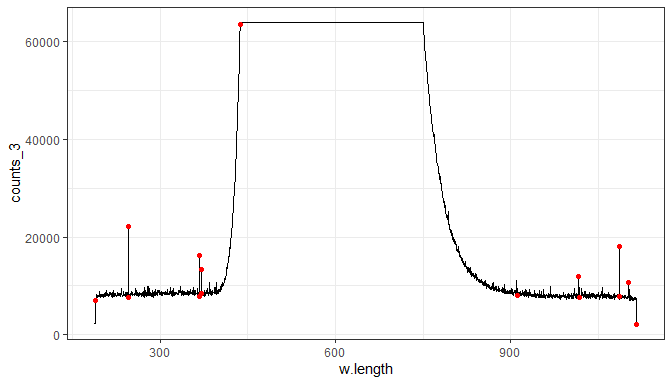``````ggplot(despike(white_led.raw_spct, z.threshold = 8, max.spike.width = 7),
aes(w.length, counts_3)) +
geom_line()``````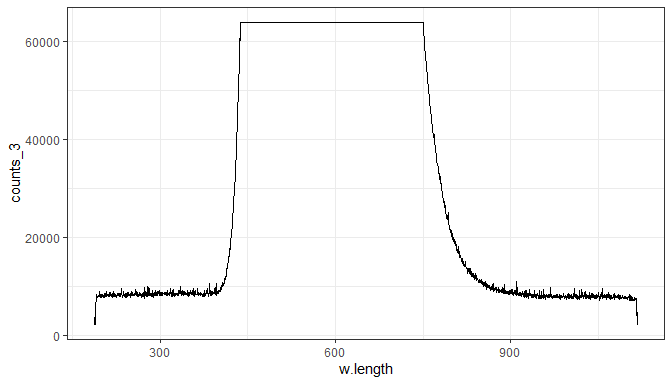## Color from wavelength

This stat calculates the colour corresponding to each \(x\)-value (assumed expressed in nanometres) and adds it to the data. It does not summarize the data like `stat_summary()` nor does it subset the data like `stat_peaks`, consequently the plot does not require any additional geom to have all observations plotted. It sets both color and fill aesthetics to a suitable default.

``````ggplot(sun.spct) +
stat_color() + scale_color_identity()``````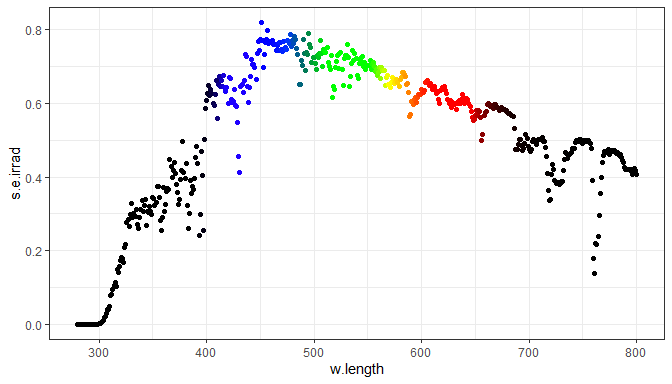All statistics that generate color definitions from wavelengths or wavebands have a parameter, `chroma.type` to which can be used to select the color matching function or color coordinates to be used. If we use chromaticity coordinates, `"CC"`, instead of the default color matching fucntion, `"CMF"`, the apparent luminance is not taken into account, only the hue.

``````ggplot(sun.spct) +
stat_color(chroma.type = "CC") + scale_color_identity()``````We here show pseudo honey-bee vision colors. Bees have trichromic vision, but see green, blue and ultraviolet (GBU) instead of red, green and blue (RGB). The luminance is matched to wavelengths, but the colors shifted so that green becomes red, blue becomes green, and ultraviolet becomes blue.

``````ggplot(clip_wl(sun.spct)) +
stat_color(chroma.type = beesxyzCMF.spct) + scale_color_identity()``````By use of a filled shape and adding a black border by overriding the default color aesthetic and over-plotting these points on top of a line, we obtain a better separation from the background.

``````ggplot(sun.spct) +
geom_line() +
stat_color(shape = 21, color = "black") +
scale_fill_identity()``````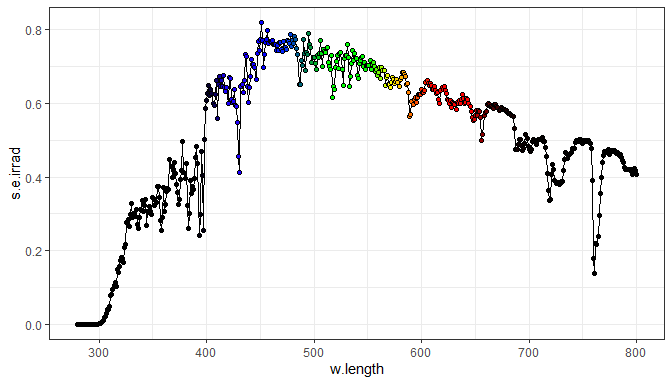With a trick using many narrow bars we can fill the area under the line with a the calculated colours. This works satisfactorily as the data set has a small wavelength step, as in this case we are using a bar of uniform colour for each wavelength value in the data set.

``````ggplot(sun.spct) +
stat_color(geom = "bar") +
geom_line(color = "black") +
geom_point(shape = 21, color = "black", stroke = 1.2, fill = "white") +
scale_fill_identity() +
scale_color_identity() +
theme_bw()``````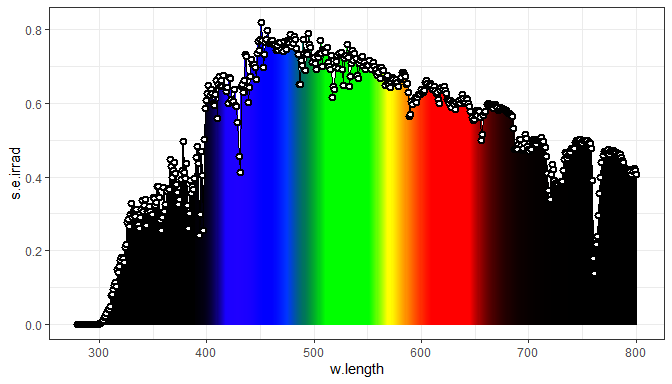``````ggplot(sun.spct) +
stat_color(geom = "bar", chroma.type = beesxyzCMF.spct) +
geom_line(color = "black") +
geom_point(shape = 21, color = "black", stroke = 1.2, fill = "white") +
scale_fill_identity() +
scale_color_identity() +
theme_bw()``````As final example we demonstrate a plot with facets and shape based groups.

``````ggplot(two_suns.spct) + aes(shape = spct.idx) +
stat_color() + scale_color_identity() +
geom_line() +
facet_grid(spct.idx~., scales = "free_y")``````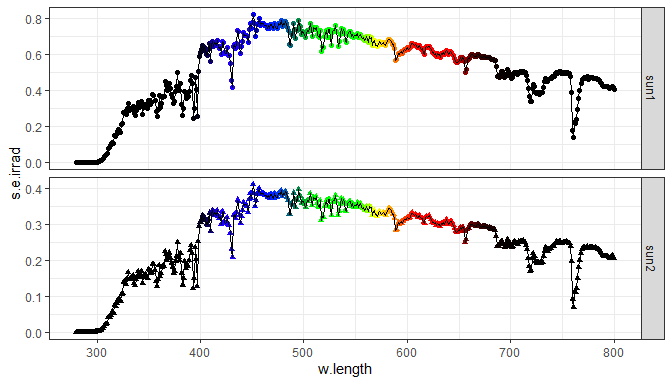## Averages and similar summaries

Our summary statistics are quite different to `ggplot2`s `stat_summary()`. One could criticize that they calculates summaries using a grouping that is not based on a ggplot aesthetic. This is a deviation from the grammar of graphics but allows the calculation of summaries for an arbitrary region of the range of \(x\)-values in the spectral data.

### Summaries producing graphical elements

Three statistics generate only graphic output. First we demonstrate `stat_wb_box()` that produces a filled box for each waveband, filled with the color corresponding to the waveband.

``````ggplot(sun.spct) + geom_line() +
stat_wb_box(w.band = VIS_bands(), color = "white") +
scale_fill_identity()``````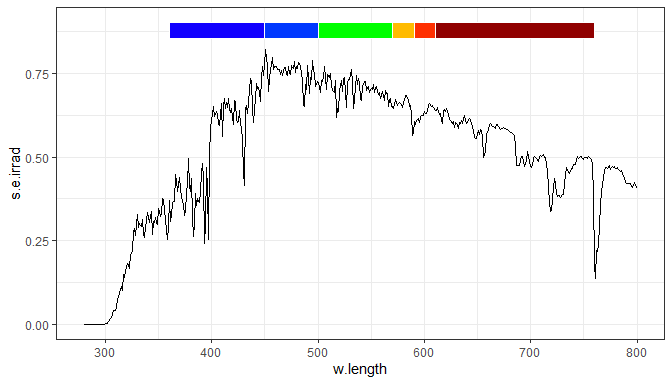The statistics `stat_wb_column` outputs a column for each waveband, with an area equal to the integral for the corresponding region of the spectrum.

``````ggplot(sun.spct) + stat_wb_column(w.band = VIS_bands()) + geom_line() +
scale_fill_identity()``````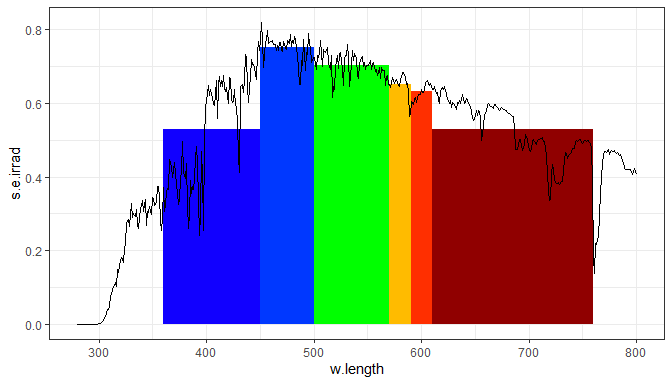The statistic `stat_wd_hbar` outputs a horizontal bar showing the mean spectral y-value for each waveband.

``````ggplot(sun.spct) + geom_line() +
stat_wb_hbar(w.band = VIS_bands(), size = 1.2) +
scale_color_identity()``````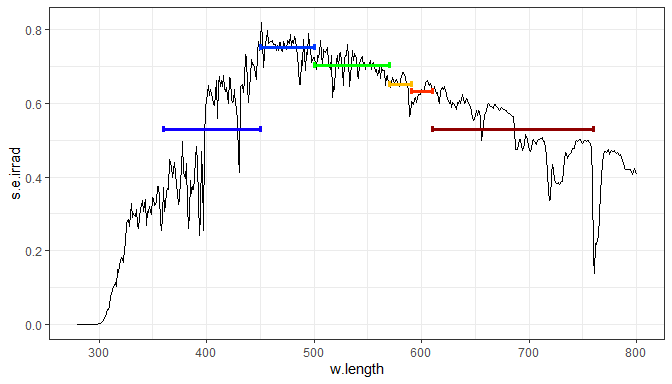These graphical summaries are frequently used together with text and label elements with names or numerical summaries.

### Name labels

``````ggplot(sun.spct) + geom_line() +
stat_wb_box(w.band = PAR(), color = "white", ypos.fixed = 0.85) +
stat_wb_label(w.band = PAR(), ypos.fixed = 0.85) +
scale_fill_identity() + scale_color_identity()``````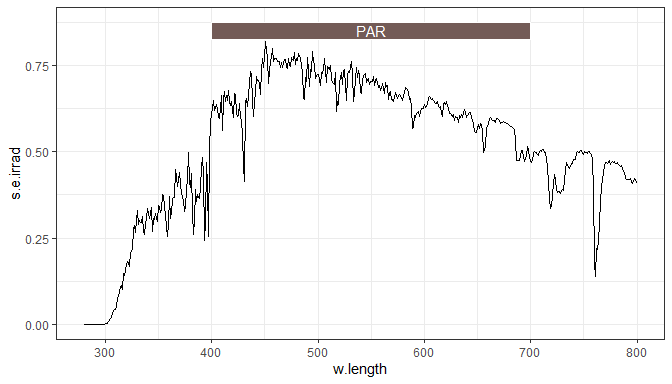### Summaries by wavelength range

The summary is calculated for a range of wavelengths, and the `range` argument defaults to the range of wavelengths in each group defined by other aesthetics. The default geom is `"text"`.

``ggplot(sun.spct) + geom_line() + stat_wl_summary()``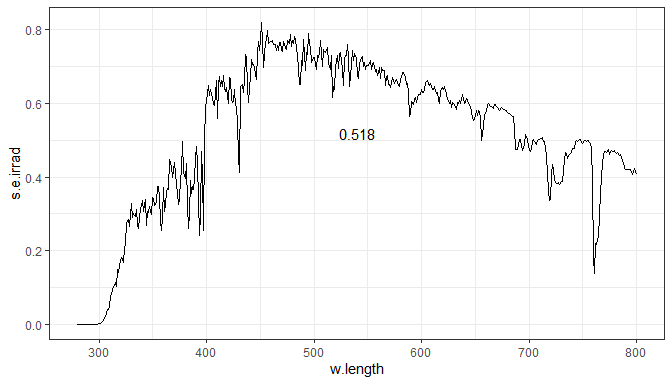We can optionally supply an argument for `range` to limit the summary to a certain part of the spectrum, in which case the use of the default `"text"` geom is misleading. We add the line last so that it is drawn on top of the rectangle.

``````ggplot(sun.spct) +
stat_wl_summary(range = c(300,350), geom = "rect") +
geom_line()``````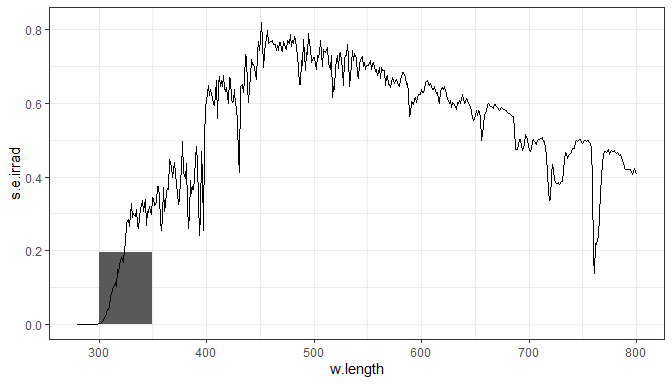Here we show how to add horizontal line and label for the overall mean, by adding the same stat twice, using different values for `geom`.

``````ggplot(sun.spct) +
geom_line() +
stat_wl_summary(geom = "hline", color = "red") +
stat_wl_summary(label.fmt = "Mean = %.3g", color = "red", vjust = -0.3)``````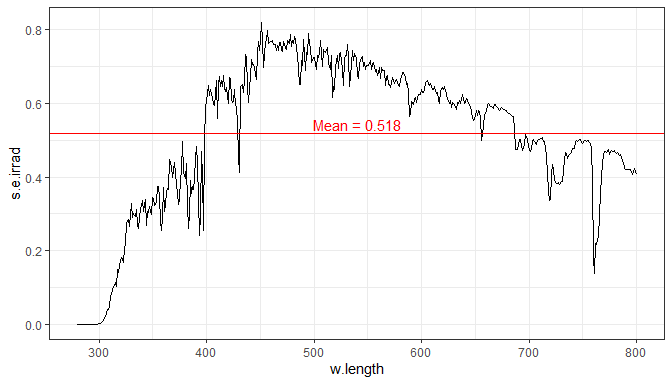Or we can add the aesthetic twice with two different geoms to get the value plotted as a rectangular area and the value as formatted text. We use `vjust` to move the text above the end of the bar, instead of it being centred on the value itself.

``````ggplot(sun.spct) +
stat_wl_summary(range = c(400,500), geom = "rect", alpha = 0.2, fill = color_of(450)) +
stat_wl_summary(range = c(400,500), label.fmt = "Mean = %.3g", vjust = -0.3, geom = "text") +
geom_line()``````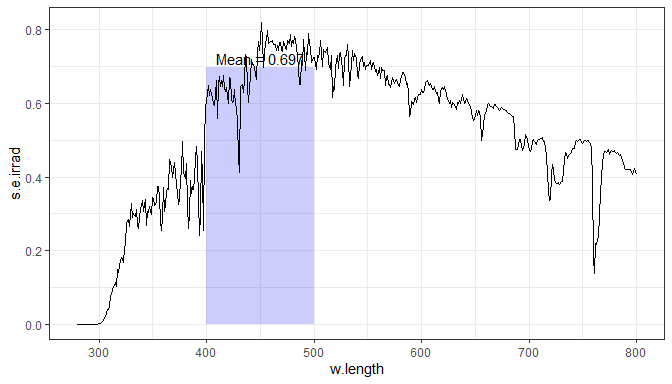An example using the `color` aesthetic for grouping and moving the text label down. Setting

``````ggplot(two_suns.spct) + aes(color = spct.idx) +
geom_line() +
stat_wl_summary(geom = "hline") +
stat_wl_summary(label.fmt = "Mean = %.3g", vjust = 1.2, show.legend = FALSE) +
facet_grid(spct.idx~.)``````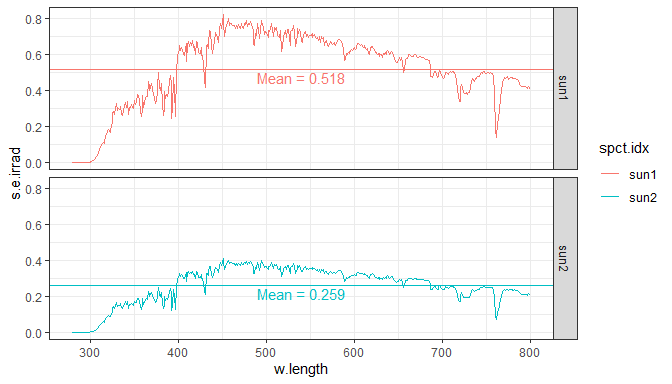Same example as above but using a free scale for \(y\), still working as expected.

``````ggplot(two_suns.spct) + aes(color = spct.idx) +
geom_line() +
stat_wl_summary(geom = "hline") +
stat_wl_summary(label.fmt = "Mean = %.3g", vjust = 1.2, show.legend = FALSE) +
facet_grid(spct.idx~., scales = "free_y")``````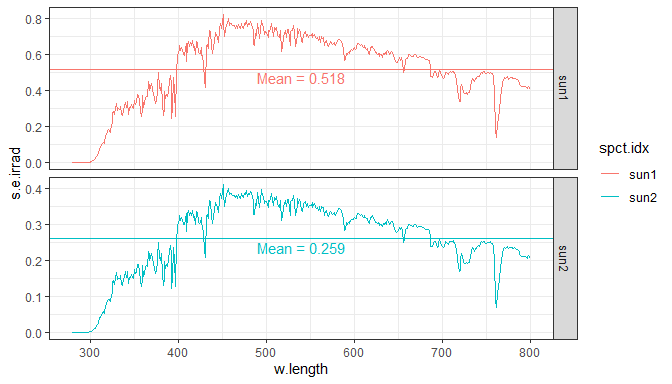### Means by waveband

Our `stat_wb_mean()` is quite different to `ggplot2`s `stat_summary()`. One could criticize that it calculates summaries using a grouping that is not based on a ggplot aesthetic. This is a deviation from the grammar of graphics but allows the calculation of summaries for an arbitrary waveband of the spectrum based on `photobiology::waveband` objects, or lists of such objects. In contrast to `stat_wl_summary()` this allows the use of several ranges, and also of different weighting functions. This function returns both mean and total values for each waveband. It differs from `stat_wb_total()` only in the default aesthetics set.

The first example uses a `waveband` object created on-the-fly and defining a range of wavelengths.

``````ggplot(sun.spct) +
geom_line() +
stat_wb_hbar(w.band = PAR(), size = 1.3) +
stat_wb_mean(aes(color = ..wb.color..), w.band = PAR(), ypos.mult = 0.95) +
scale_color_identity() +
scale_fill_identity() +
theme_bw()``````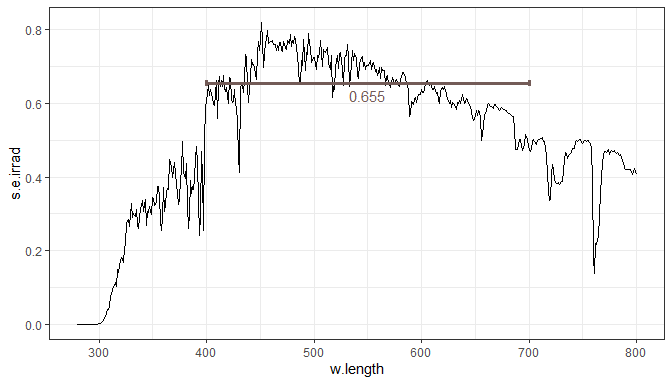If a numeric vector or a spectrum is supplied as argument to `waveband`, its range is calculated and used to construct a temporary `waveband` object.

``````ggplot(sun.spct) +
stat_wb_hbar(w.band = c(400,500), size = 1.2) +
stat_wb_mean(aes(color = ..wb.color..),
w.band = c(400,500), ypos.mult = 0.95) +
geom_line() +
scale_color_identity() +
scale_fill_identity() +
theme_bw()``````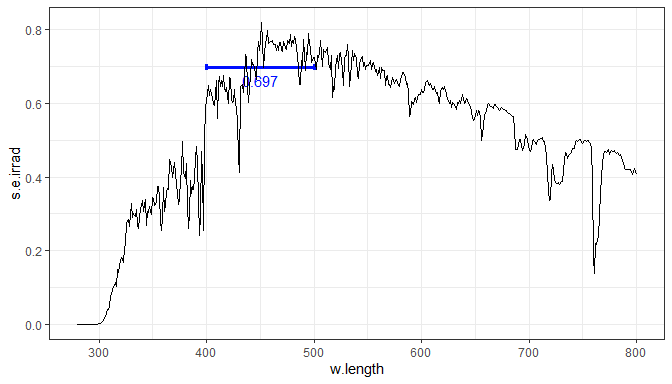Lists of wavebands, either user-defined or as in this case using a list constructor can be also used.

``````ggplot(sun.spct) +
geom_line() +
stat_wb_hbar(w.band = list(Blue(), Red()), size = 1.2) +
stat_wb_mean(aes(color = ..wb.color..),
w.band = list(Blue(), Red()), ypos.mult = 0.95,
hjust = 1, angle = 90) +
scale_color_identity() +
scale_fill_identity() +
theme_bw()``````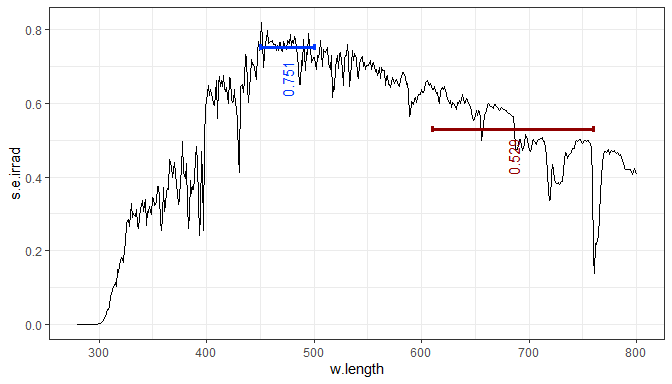### Totals by waveband

Our `stat_wb_total()` is quite different to `ggplot2`s `stat_summary()`. One could criticize that it calculates summaries using a grouping that is not based on a ggplot aesthetic. This is a deviation from the grammar of graphics but allows the calculation of summaries for an arbitrary waveband of the spectrum based on `photobiology::waveband` objects, or lists of such objects. In contrast to `stat_wl_summary()` this allows the use of several ranges, and also of different weighting functions. This function returns both mean and total values for each waveband. It differs from `stat_wb_mean()` only in the default aesthetics set.

The first example uses a `waveband` object created on-the-fly and defining a range of wavelengths.

``````ggplot(sun.spct) +
stat_wb_box(w.band = PAR()) +
stat_wb_total(w.band = PAR()) +
geom_line() +
scale_color_identity() +
scale_fill_identity() +
theme_bw()``````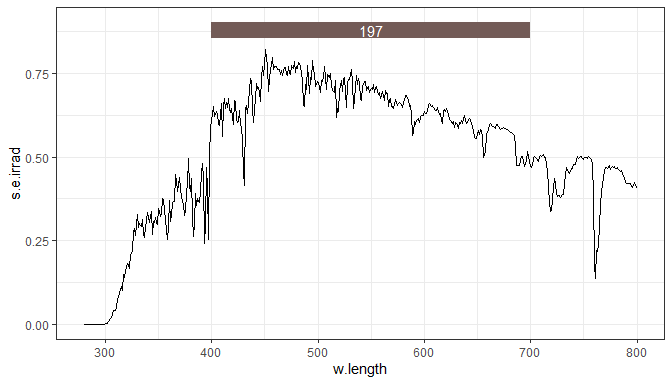If a numeric vector or a spectrum is supplied as argument to `waveband`, its range is calculated and used to construct a temporary `waveband` object.

``````ggplot(sun.spct) +
stat_wb_box(w.band = c(400,500)) +
stat_wb_total(w.band = c(400,500)) +
geom_line() +
scale_color_identity() +
scale_fill_identity() +
theme_bw()``````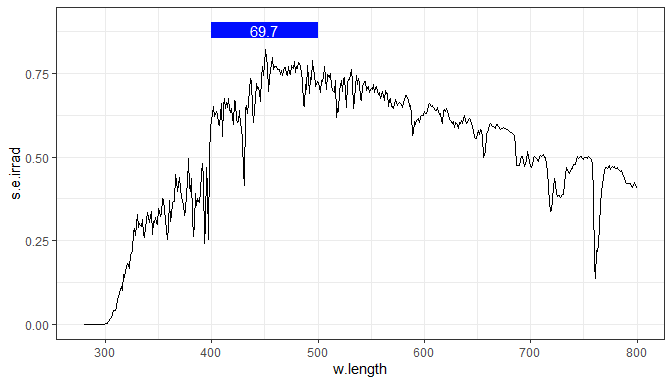Lists of wavebands, either user-defined or as in this case using constructor defined in package `photobiologyWavebands` can be also used. In the case of totals, areas represent them graphically in a very useful way.

``````ggplot(sun.spct * yellow_gel.spct) +
stat_wb_box(w.band = Plant_bands(), color = "white", ypos.fixed = 0.7) +
stat_wb_column(w.band = Plant_bands(), color = "white", alpha = 0.5) +
stat_wb_mean(w.band = Plant_bands(), label.fmt = "%1.2f",
ypos.fixed = 0.7, size = 2) +
geom_line() +
scale_fill_identity() + scale_color_identity() +
theme_bw()``````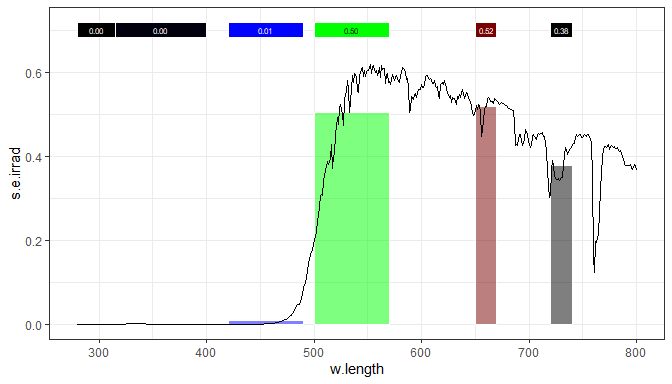### Irradiances by waveband

The _stat_s in previous sections can be used for non-weighed irradiances, but not for BSWFs. These `irrad` _stat_s have additional formal parameters for passing metadata, and use method `photobiology::irrad()` internally on a `photobiology::source_spct` object constructed from the data for \(x\) and \(y\) aesthetics. Function `stat_wb_sirrad()` is equivalent to `stat_wb_mean()` and function `stat_wb_irrad()` is equivalent to `stat_wb_total()` following the same naming convention as in package `photobiology`.

``````ggplot(sun.spct) +
stat_wb_box(w.band = PAR()) +
stat_wb_irrad(w.band = PAR(), unit.in = "energy", time.unit = "second") +
geom_line() +
scale_color_identity() +
scale_fill_identity() +
theme_bw()````````````ggplot(sun.spct, unit.out = "photon") +
stat_wb_box(w.band = PAR()) +
stat_wb_irrad(w.band = PAR(),
unit.in = "photon", time.unit = "second",
aes(label = sprintf("%s = %.3g", ..wb.name.., ..wb.yint.. * 1e6))) +
geom_line() +
scale_color_identity() +
scale_fill_identity() +
theme_bw()``````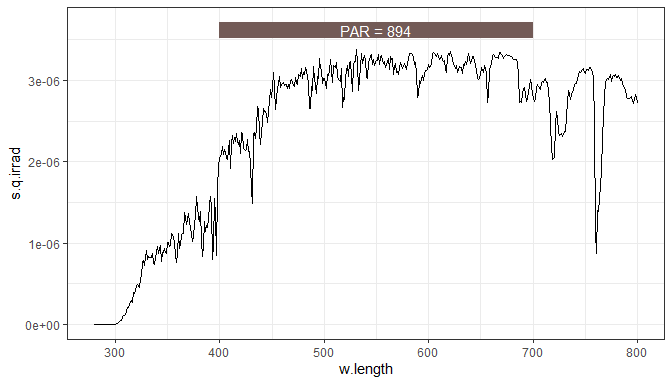Also following the same naming convention as in package `photobiology`, `e` and `q` versions of these functions default to energy and photon based quantities to `"seconds"` for time unit.

``````ggplot(sun.spct) +
stat_wb_box(w.band = PAR()) +
stat_wb_e_irrad(w.band = PAR()) +
geom_line() +
scale_color_identity() +
scale_fill_identity() +
theme_bw()``````We can also use `waveband` objects describing biological spectral weighting functions (BSWFs), such as CIE’s erythema function.

``````ggplot(sun.spct) +
stat_wb_box(w.band = CIE()) +
stat_wb_e_irrad(w.band = CIE()) +
geom_line() +
scale_color_identity() +
scale_fill_identity() +
theme_bw()``````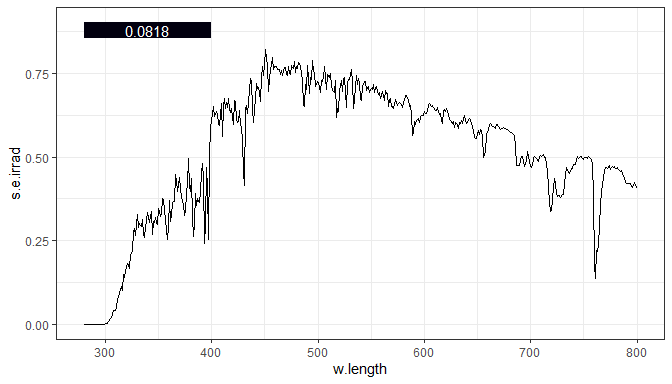For daily data we need to override the default time unit.

``````ggplot(sun.daily.spct) +
stat_wb_box(w.band = CIE()) +
stat_wb_e_irrad(w.band = CIE(), time.unit = "day", label.mult = 1e-3) +
geom_line() +
scale_color_identity() +
scale_fill_identity() +
theme_bw()``````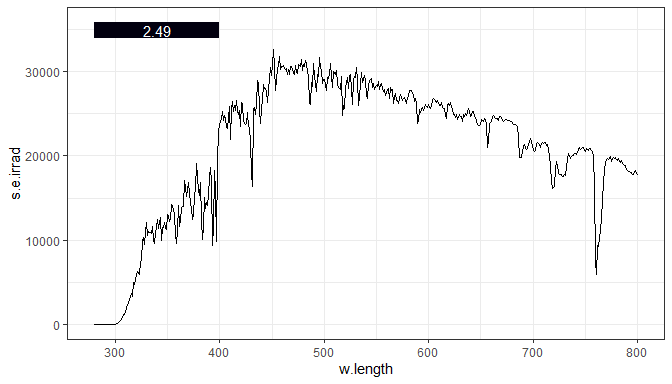Here we pass a list of `waveband` objects. And add areas to facilitate comparisons as irradiances are integral over wavelengths.

``````ggplot(sun.spct, unit.out = "photon") +
stat_wb_box(w.band = VIS_bands(), color = "black") +
stat_wb_column(w.band = VIS_bands(), color = NA, alpha = 0.5) +
stat_wb_q_irrad(w.band = VIS_bands(), label.mult = 1e6, size = 2) +
geom_line() +
scale_color_identity() +
scale_fill_identity() +
theme_bw()``````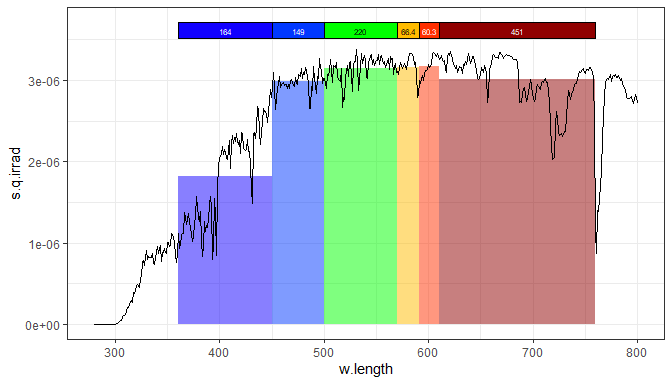### Spectral irradiances by waveband

Spectral irradiances are equivalent to means, and can be best represented graphically as horizontal bars.

``````ggplot(sun.spct) +
geom_line() +
stat_wb_hbar(w.band = PAR(), size = 1.4) +
stat_wb_e_sirrad(aes(color = ..wb.color..),
w.band = PAR(), ypos.mult = 0.95) +
scale_color_identity() +
scale_fill_identity() +
theme_bw()````````````ggplot(sun.spct, unit.out = "photon") +
stat_wb_column(w.band = PAR(), alpha = 0.8) +
stat_wb_q_sirrad(w.band = PAR(),
mapping =
aes(label = sprintf("Total %s = %.3g",
..wb.name.., ..wb.yint.. * 1e6)),
ypos.mult = 0.55) +
stat_wb_q_sirrad(w.band = PAR(),
mapping =
aes(label = sprintf("Mean %s = %.3g",
..wb.name.., ..wb.ymean.. * 1e6)),
ypos.mult = 0.45) +
geom_line() +
scale_color_identity() +
scale_fill_identity() +
theme_bw()``````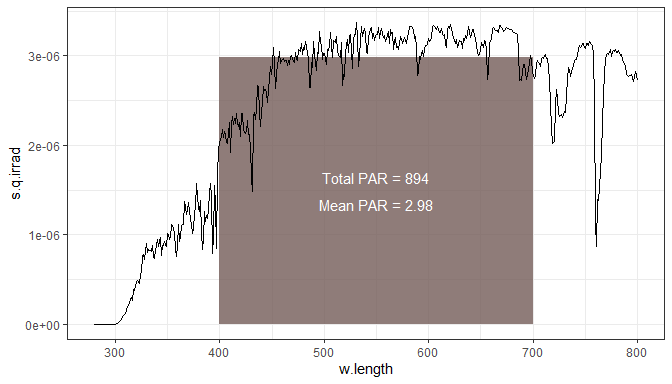``````ggplot(sun.spct) +
stat_wb_box(w.band = waveband(CIE()), ypos.fixed = 0.85) +
stat_wb_e_sirrad(w.band = CIE(), ypos.fixed = 0.85) +
geom_line() +
scale_color_identity() +
scale_fill_identity() +
theme_bw()``````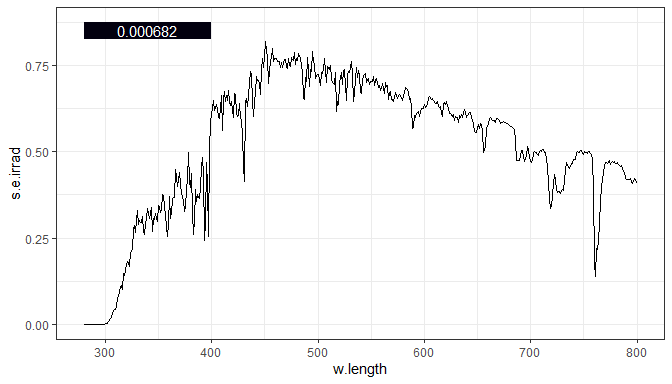``````ggplot(sun.daily.spct) +
stat_wb_box(w.band = waveband(CIE()), ypos.fixed = 34e3) +
stat_wb_e_sirrad(w.band = CIE(),
label.fmt = "%.2g kj / day",
time.unit = "day",
ypos.fixed = 34e3) +
geom_line() +
scale_color_identity() +
scale_fill_identity() +
theme_bw()``````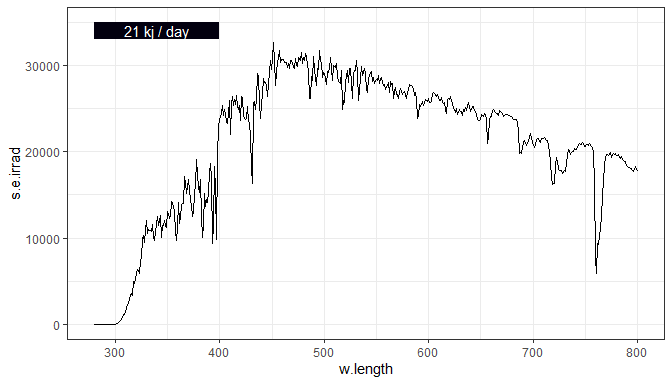``````my.bands <- split_bands(c(300,800), length.out = 10)
ggplot(sun.spct, unit.out = "photon") +
stat_wb_hbar(w.band = my.bands, size = 1.4) +
stat_wb_q_sirrad(geom = "label", w.band = my.bands,
size = 2.5, ypos.fixed = 3.5e-6) +
geom_line() +
scale_color_identity() +
scale_fill_identity() +
theme_bw()``````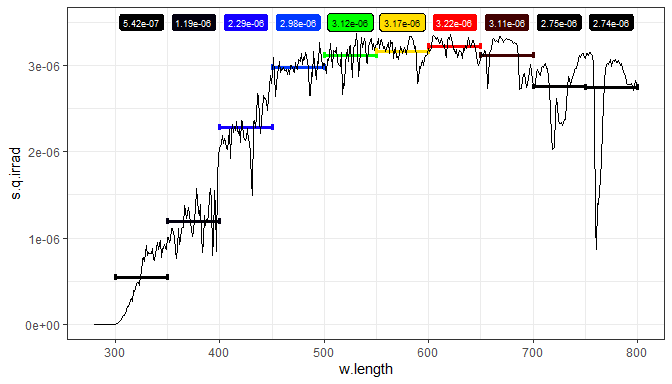### Irradiances by waveband

``````ggplot(sun.spct) +
stat_wb_column(w.band = VIS_bands(), alpha = 0.5) +
stat_wb_e_irrad(w.band = VIS_bands(), angle = 90,
ypos.fixed = 0.05, hjust = 0,
aes(label = paste(..wb.name.., ..y.label.., sep = " = "))) +
geom_line() +
scale_color_identity() +
scale_fill_identity() +
theme_bw()``````